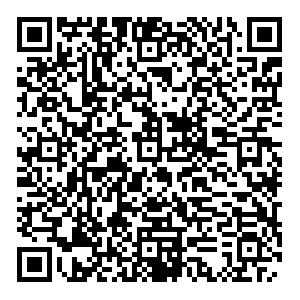# Strong cosmic censorship for a scalar field in an Einstein-Maxwell-Gauss-Bonnet-de Sitter black hole

• It has been shown that the Christodoulou version of the strong cosmic censorship (SCC) conjecture can be violated for a scalar field in a near-extremal Reissner-Nordstrom-de Sitter black hole. In this paper, we investigate the effects of higher derivative corrections to the Einstein-Hilbert action on the validity of SCC, by considering a neutral massless scalar perturbation in $5$- and $6$-dimensional Einstein-Maxwell-Gauss-Bonnet-de Sitter black holes. Our numerical results show that the higher derivative term plays a different role in the $d = 5$case than it does in the $d = 6$case. For $d = 5$, the SCC violation region increases as the strength of the higher derivative term increases. For $d = 6$, the SCC violation region first increases and then decreases as the higher derivative correction becomes stronger, and SCC can always be restored for a black hole with a fixed charge ratio when the higher derivative correction is strong enough. Finally, we find that the $C^{2}$version of SCC is respected in the $d = 6$case, but can be violated in some near-extremal regimes in the $d = 5$case.
••Get Citation
Qingyu Gan, Peng Wang, Houwen Wu and Haitang Yang. Strong cosmic censorship for a scalar field in an Einstein-Maxwell-Gauss-Bonnet-de Sitter black hole[J]. Chinese Physics C.
Qingyu Gan, Peng Wang, Houwen Wu and Haitang Yang. Strong cosmic censorship for a scalar field in an Einstein-Maxwell-Gauss-Bonnet-de Sitter black hole[J]. Chinese Physics C.Milestone
Revised: 2020-09-30
Article Metric

Article Views(25)
Cited by(0)
Policy on re-use
To reuse of subscription content published by CPC, the users need to request permission from CPC, unless the content was published under an Open Access license which automatically permits that type of reuse.
###### 通讯作者: 陈斌, bchen63@163.com
• 1.

沈阳化工大学材料科学与工程学院 沈阳 110142

Title:
Email:

## Strong cosmic censorship for a scalar field in an Einstein-Maxwell-Gauss-Bonnet-de Sitter black hole

• Center for Theoretical Physics, College of Physics, Sichuan University, Chengdu 610064, China

Abstract: It has been shown that the Christodoulou version of the strong cosmic censorship (SCC) conjecture can be violated for a scalar field in a near-extremal Reissner-Nordstrom-de Sitter black hole. In this paper, we investigate the effects of higher derivative corrections to the Einstein-Hilbert action on the validity of SCC, by considering a neutral massless scalar perturbation in $5$- and $6$-dimensional Einstein-Maxwell-Gauss-Bonnet-de Sitter black holes. Our numerical results show that the higher derivative term plays a different role in the $d = 5$case than it does in the $d = 6$case. For $d = 5$, the SCC violation region increases as the strength of the higher derivative term increases. For $d = 6$, the SCC violation region first increases and then decreases as the higher derivative correction becomes stronger, and SCC can always be restored for a black hole with a fixed charge ratio when the higher derivative correction is strong enough. Finally, we find that the $C^{2}$version of SCC is respected in the $d = 6$case, but can be violated in some near-extremal regimes in the $d = 5$case.

### HTML关注分享DownLoad:  Full-Size Img  PowerPoint